# 「Game Tricks」地形纹理合并

Posted by Candycat on November 28, 2016

# 方法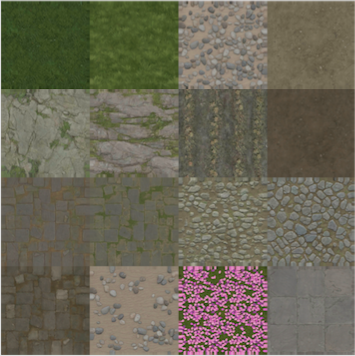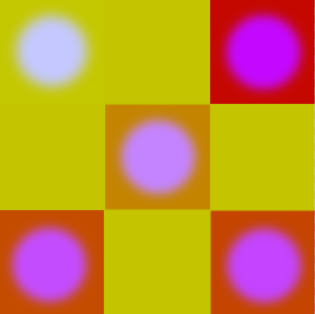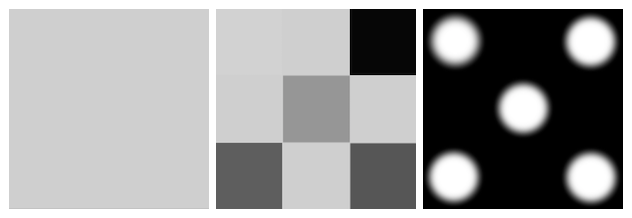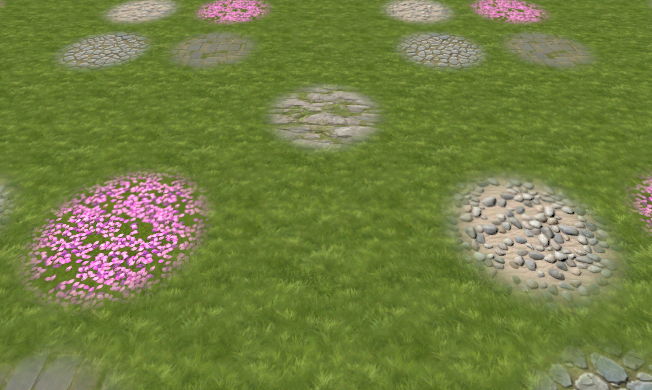## 地形纹理的索引

$f = \frac{x}{16} + \frac{y}{256}$

$x = floor(16f)\\y = floor(256f) - 16x$

1
2
3
4
5
6
7
8
9
10
11
float2 encodedIndices = tex2D(_BlendTex, i.uv).xy;

float2 twoVerticalIndices = floor((encodedIndices * 16.0));
float2 twoHorizontalIndices = (floor((encodedIndices * 256.0)) - (16.0 * twoVerticalIndices));

float4 decodedIndices;
decodedIndices.x = twoHorizontalIndices.x;
decodedIndices.y = twoVerticalIndices.x;
decodedIndices.z = twoHorizontalIndices.y;
decodedIndices.w = twoVerticalIndices.y;
decodedIndices = floor(decodedIndices/4)/4;


decodedIndices就是0~3的整数索引值除以4的结果，即该种纹理在BlockMainTex中的起始值。拿图中樱花那个block举例，它对应的xy值是（0，8）（由于xy的范围是0~15，而图片索引范围是0~3，所以要乘以4），所以在BlendTex中的颜色就是8/256。

## 纹理采样

1
2
3
4
5
float2 worldScale = (worldPos.xz * _BlockScale);
float2 worldUv = 0.234375 * frac(worldScale) + 0.0078125; // 0.0078125 ~ 0.2421875, the range of a block

float2 uv0 = worldUv.xy + decodedIndices.xy;
float2 uv1 = worldUv.xy + decodedIndices.zw;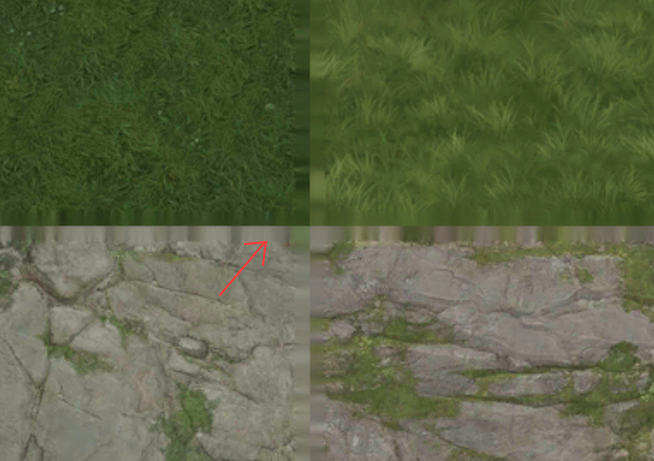## 处理接缝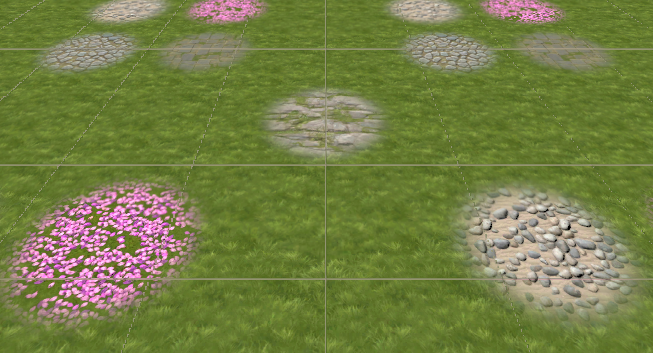1
2
3
4
5
6
7
8
9
10
11
12
13
14
float blendRatio = tex2D(_BlendTex, i.uv).z;

float2 worldScale = (worldPos.xz * _BlockScale);
float2 worldUv = 0.234375 * frac(worldScale) + 0.0078125;
float2 dx = clamp(0.234375 * ddx(worldScale), -0.0078125, 0.0078125);
float2 dy = clamp(0.234375 * ddy(worldScale), -0.0078125, 0.0078125);

float2 uv0 = worldUv.xy + decodedIndices.xy;
float2 uv1 = worldUv.xy + decodedIndices.zw;
// Sample the two texture
float4 col0 = tex2D(_BlockMainTex, uv0, dx, dy);
float4 col1 = tex2D(_BlockMainTex, uv1, dx, dy);
// Blend the two textures
float4 col = lerp(col0, col1, blendRatio);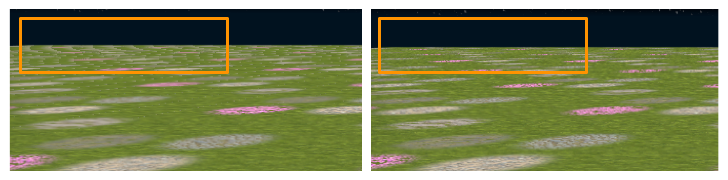# 写在最后

1
2
3
4
5
6
// Sample the two normal
fixed3 norm0 = UnpackNormal(tex2D(_BlockNormalTex, uv0, dx, dy));
fixed3 norm1 = UnpackNormal(tex2D(_BlockNormalTex, uv1, dx, dy));
// Blend the two normals
fixed3 norm = lerp(norm0, norm1, blendRatio);
norm = normalize(norm);